Imaginary numbers

# 1  Introduction

We are familiar with finding the square root of a positive number i.e.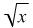where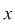is greater than or equal to zero (usually abbreviated by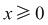). There are two solutions to every problem of this type i.e.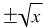. However, whenis negative (written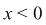) there is no real number which, when multiplied by itself, gives an answer which is a negative number. In order to overcome this gap in the number system and enable a solution to be found to the equation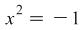, the symbol ‘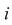’ is used to represent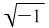. (In engineering, an alternative, ‘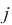’ is used to avoid confusion with the symbol representing current.) The symbol(or) is treated in exactly the same way as any other number. All of the normal arithmetical operations can be carried out using it. Multiplication ofby a real number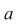i.e.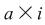is written as either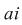or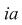. Any real multiple ofis called an imaginary number.

## 1.1  Examples

1. Multiplyby 3.

Solution:

Multiplication by 3 is equivalent to addingto itself twice i.e.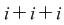. This is written as either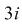or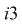.

2. Divideby 6.

Solution:

Sinceis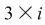, this can be re-written as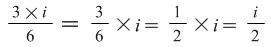.

# 2  Powers of an imaginary number

This is performed in the same way as with real numbers, i.e.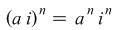.

## 2.1  Example

Evaluate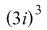Solution: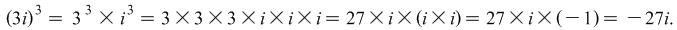# 3  Square roots of a negative number

In the same way that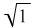has two solutions i.e.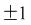, soalso has two solutions i.e.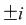. This can be shown by multiplying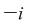by itself: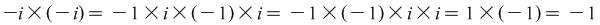.

This result can be extended and generalised to give the square roots of any negative number.

## 3.1  Example

Find the square roots of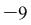.

Solution: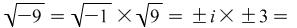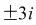.